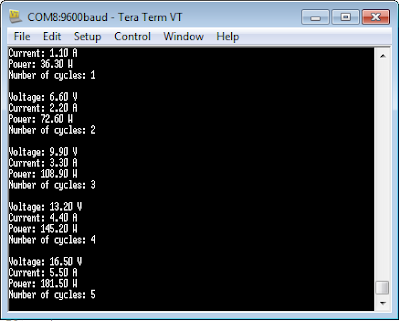## Introduction

A queue is a fundamental way to establish InterProcess Communications (IPC). Queues are used to send messages between tasks, and between interrupts and tasks. A First In First Out (FIFO) approach is used in buffers with new data being sent to the back of the queue. If task A is sending a number of messages to task B, then the first message will be placed in the back of the queue. This is what task B will read from first. Then message 1 will be read from the queue and message 2 can enter the back of the queue.

## Run, Record, Review

The rtos_queue program was run which outputs a fake voltage and current reading. The values are being passed as messages using a pointer.  The queue can take up to 16 messages. The memory pool is used t allocate fixed sized data blocks to store data in.

The code sets up a structure of voltage, current, and a counter. There is a thread called "send_thread" which cycles from 0 and this is used to generate fake voltage and current outputs. The counter is simply the value of the cycle (i). This will keep looping as long as the board has power.
The main thread gets the message from the queue if there is a message in it. This then uses printf statements to print the values of voltage, current, and the counter to the user. It then frees up the memory pool.

The code was reviewed and another value was added to the original structure as follows:

typedef struct {
float    voltage;   /* AD result of measured voltage */
float    current;   /* AD result of measured current */
float    power;     /* AD result of measured power   */
uint32_t counter;   /* A counter value               */
} message_t;

The power variable was initialised as  a float within the structure. Since P = VI, the value that the index is multiplied by is the voltage and current. Since voltage was multiplied by 33 and current 11, the power was multiplied by 363. The code was run using the changes and the output was as follows:Queue output with revisions made

The queue first passes the value of voltage, then current, then power and finally the number of cycles. It can be seen that the first message in the queue is also the first one to be read into the next function. This is a very simple way of safely passing messages from one thread to another in an RTOS.  The C++ code is as below:

#include "mbed.h"

typedef struct {
float    voltage;   /* AD result of measured voltage */
float    current;   /* AD result of measured current */
float    power;     /* AD result of measured power */
uint32_t counter;   /* A counter value               */
} message_t;

MemoryPool mpool;
Queue queue;

/* Send Thread */
void send_thread (void) {
uint32_t i = 0;
while (true) {
i++; // fake data update
message_t *message = mpool.alloc();
message->voltage = (i * 0.1) * 33;
message->current = (i * 0.1) * 11;
message->power   = (i * 0.1) * 363;
message->counter = i;
queue.put(message);
wait(1);
}
}

int main (void) {

while (true) {
osEvent evt = queue.get();
if (evt.status == osEventMessage) {
message_t *message = (message_t*)evt.value.p;
printf("\nVoltage: %.2f V\n\r"   , message->voltage);
printf("Current: %.2f A\n\r"     , message->current);
printf("Power: %.2f W\n\r"       , message->power);
printf("Number of cycles: %u\n\r", message->counter);

mpool.free(message);
}
}
}